Published: 30 September 2016

# Effects of thermal aging of laminated rubber bearing on seismic performance of bridges

Ju Oh1
Jin Ho Kim2
Hwa Cho Yi3
1Korean Intellectual Property Office, Daejeon, Korea
2, 3Department of Mechanical Engineering, Yeungnam University, Gyeongsan, Korea
Corresponding Author:
Hwa Cho Yi
Views 527

#### Abstract

The dynamic characteristics of laminated rubber bearings, which are used as isolator, are dependent on the main rubber's dynamic behaviors and nonlinear properties. Rubber materials tend to undergo an aging process under the influence of mechanical or environmental factors, so they inevitably end up facing damage. A main cause of aging like this is known to be oxidization, which occurs through the heat of reaction at high temperatures. Accordingly, in this study an accelerated thermal aging test was carried out in order to compare the characteristic values of the bearings before and after thermal aging occurs. As a result of this experiment, it was found that a thermal aging phenomenon could have some effects on shear stiffness, energy absorption, and equivalent damping coefficients of the bearings. Furthermore, a deterioration in the dynamic properties of the laminated rubber bearings caused by the thermal aging was applied to an actual bridge and then the effects of such thermal aging on the seismic performance of the bridge were also compared and analyzed based on numerical analysis. As a result of this analysis, it was found that the changes in the basic properties of the natural rubber bearings caused by the thermal aging bring only a minor effect on the seismic performance of bridges.

## 1. Introduction

The laminated rubber bearing is a type of seismic isolation bearing that a reinforcing steel plate is laminated between rubbers in form of layer so that it prevents the buckling behavior of rubber for vertical load and maintains high vertical stiffness while showing the flexibility of rubber in the horizontal direction. When carrying out the seismic isolation design using this laminated rubber bearing, the shear stiffness and energy dissipation effect of bearing depend on the nonlinear property of rubber material used as the main material at the time of dynamic behavior. In case of laminated rubber bearing, the ageing process of rubber occurs due to several mechanical and environmental effects and damage occurs to rubber inevitably after all. Generally, when rubber has been exposed to the air for a long period of time, it began to harden so that a crack or stickiness occurs on the rubber surface, and it is called aging. The leading causes of aging are the oxidation process due to oxygen in air, heat, ozone and metallic salt, and these elements accelerate the aging process of rubber. When the temperature increases due to heat among the causes of rubber aging, the heat of reaction occurs, accelerating the oxidation process. Fitzgrald et al. (1992) revealed the correlation with heat and cyclic load such that when the cyclic stress applied to rubber, the physical characteristics of elastic body decreased as the temperature was higher . Also, Choi et al. studied the effects of molecular structure changes of rubber according to thermal aging and revealed that as the thermal aging time increased, the crosslink density increased due to continuous rubber vulcanization increase . And, the shear failure test of rubber specimen was performed by changing the temperature from 60 °C to 120 °C while exposing the natural rubber to air and salt water. The result showed that hot air in the atmosphere had a higher effect than salt water . The thermal aging of laminated rubber bearing is related to environments such as high temperature, and it is possible that another destructive mechanism may occur accordingly. If the laminated rubber bearing installed on bridges and buildings has been exposed to deterioration environments for a long time so that aging has occurred, uncertain vibrations such as earthquake may cause serious damage to the structures. Therefore, the dynamic characteristics of natural rubber bearing in thermal aging status is one of elements that should be considered in term of durability for designing the seismic isolation structure. Also, the effects of seismic isolation systems have been proven through many previous tests and studies so that the installation cases of seismic isolation systems such as laminated rubber bearing on bridges are increasing and many studies regarding the evaluation and improvement of seismic performance of bridges using such bearing are being carried out [4, 5]. Studies to review and predict characteristics change according to aging targeting the natural rubber beatings on an actual bridge were also carried out [6, 7]. However, there has been almost no study regarding changes in the seismic performance of the bridge due to aging of laminated rubber bearing. Therefore, in order to evaluate the thermal aging characteristics of laminated rubber bearing using natural rubber, the accelerated thermal aging test was carried out in this study, the structure analysis was performed by applying the characteristics of specimen before and after thermal aging to an actual bridge and the influence of aging of laminated rubber bearing on the seismic performance of the bridge was compared and analyzed. In this study, the seismic performance evaluation of bridge was carried out with the supplied capacity and the required capacity in non-isolated status from an actual bridge designed and applied with the laminated rubber bearing. From this evaluation, it was confirmed whether the bearing capacity was proper or not, and changes in the seismic performance of the bridge due to thermal aging were examined.

## 2. Thermal aging test and analysis of laminated rubber bearing

### 2.1. Specification of specimen

The characteristic and durability test and evaluation of specimens were carried out according to ISO22762 (2010) [8, 9]. The above standard consists of 3 parts including the test method and test and evaluation standards of seismic isolator in form of laminated rubber bearing used for protecting a bridge or building structure from an earthquake. Natural rubber has excellent elastic force and restoring force so that it has been widely used for the seismic isolator. The natural rubber bearing (NRB) having the elastic restoring force which was the inherent property of natural rubber and the lead rubber bearing (LRB) were used the specimens in this study. The diameter of specimen included the thickness of external rubber (10 mm), and the thickness of one internal laminated rubber layer was 3 mm each. 29 lamination layers for the rubber were used and the thickness of reinforcing steel plate was 3 mm. ${S}_{1}$is primary shape factor as the ratio of load area to the free surface area including one rubber layer hole, and ${S}_{2}$ is the secondary shape factor indicating the ratio of effective width to the total width of internal rubber. The detailed specifications of specimen are as shown in Table 1, and Fig. 1 shows the shape and sectional specifications of specimen. The specimens used for the thermal aging test were designed as same as the laminated rubber bearing which was actually applied to the bridges approximately 16 years ago, and the natural rubber with shear strain of 100 % and shear modulus of 0.4 MPa was used. As a result of examining the target bearing according to the current bridge design standard, the shape factor was too small so that it is unsuitable for the current design standard. It's because no design concept for the laminated rubber bearing was established in the past, so it does not satisfy the current design standard.

### 2.2. Test conditions and method

The tester used for the test of laminated rubber bearing specimen is the compression-shear tester which has the maximum vertical load of 2,000 kN and the maximum horizontal load of 500 kN. And, the maximum horizontal speed is 250 mm/sec and the maximum vertical displacement is ±200 mm. The shear characteristics of laminated rubber bearing before and after thermal aging were compared to determine the compression and shear characteristics according to thermal aging. For the aging test, the shear characteristics and extreme shear characteristics of the specimens were tested before aging, and then after aging the specimens in an aging oven for 168 hours at a temperature of 70 °C, the shear test and the extreme shear failure test were carried out. 3 specimens before and after thermal aging each were used for the ultimate shear failure test, and these specimens were not used again after the test. The specimens used in this study were 16 specimens including 8 natural rubber bearings and 8 lead rubber bearings. The specimens were divided into 4 specimens before and after thermal aging, and the extreme failure test was carried out after the compression and shear test, and the cyclic loading test was carried out using additional 1 specimen. Also, in order to reduce a manufacturing error of specimen and an error which may occur during the test, the average value was taken from the result of each test. The compression-shear test which was the basic test was carried out using the same specimens before and after thermal aging, and the extreme shear test was also carried out using the specimens before and after thermal aging separately.

Table 1Parameters of laminated rubber bearing

 Parameters Value Parameters Value Steel plate diameter, ${D}_{s}$ 259 mm Rubber thickness, ${t}_{i}$ 3 mm Inner hole diameter, ${D}_{p}$ 56 mm Rubber layer, $n$ 29 Total diameter, $D$ 279 mm Steel plate thickness, ${t}_{s}$ 3 mm 1st Shape factor, ${S}_{1}$ 21.6 2nd Shape factor, ${S}_{2}$ 2.98 Shear modulus, $G$ 0.4 MPa Here, ${S}_{1}=D-{D}_{P}/4{t}_{i},{S}_{2}=D/n{t}_{i}$

Fig. 1Cross section of laminated rubber bearing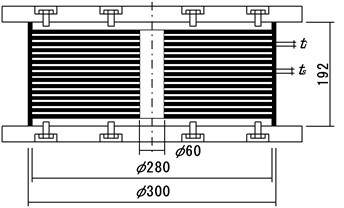### 2.3.1. Compression test

After thermal aging the laminated rubber bearing specimens (70 °C, 168 hr) according to the test conditions and carrying out the compression test, the result as shown in Table 2 was obtained. At this time, the design compressive force of lead rubber bearings was 565 kN and the design compressive force of natural rubber bearings was 490 kN, and the design compressive force ±30 % three times each was loaded on each test. As a result, in case of lead rubber bearing specimen, its compression stiffness before aging was 478 kN/mm but its compression stiffness after heat aging was 462 kN/mm, showing an approximately 3.3 % decrease. And, in case of natural rubber bearing specimen, its compression stiffness before aging was 195 kN/mm and its compression stiffness after aging was 191 kN/mm, showing an approximately 2 % decrease. The load-displacement history curve after thermal aging is as shown in Fig. 2, and the deformation curve for each type of specimen shows that the compressive force after aging increased slightly in comparison to the compressive force before aging. Also, after heat aging, the vertical displacement decreased and the slope increased. This is because the elongation percentage decreased as the stiffness of rubber increased due to thermal aging just as the rubber material specimen test result.

Fig. 2Hysteresis curve of compression test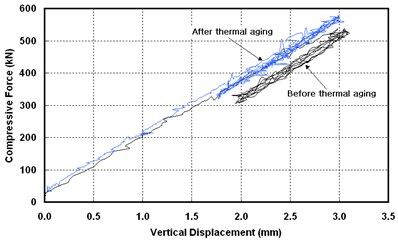a) NRB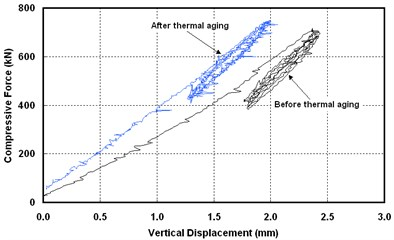b) LRB

Table 2Result of compression test

 Item Compression stiffness (kN/mm) NRB LRB Virgin 195 478 Aged 191 462 Change ratio (%) –2.05 –3.34

Table 3Result of compression-shear test

 Item Shear stiffness (kN/mm) Equivalent damping ratio (%) NRB LRB NRB LRB Virgin 0.196 0.73 7.56 35.28 Aged 0.195 0.70 7.35 35.16 Change ratio (%) –0.51 –4.10 –0.27 –0.34

### 2.3.2. Shear test

As a result of compression-shear test before and after thermal aging, the shear stiffness of lead rubber bearing was 0.73 kN/mm before aging and 0.70 kN/mm after aging, showing an approximately 4 % decrease as shown in Table 3, and the shear stiffness of natural rubber bearing was 0.196 kN/mm before aging and 0.195kN/mm after aging, showing an approximately 0.5 % decrease. And, the equivalent damping ratio of lead rubber bearing was 35.28 % before aging and 35.16 % after aging, showing an approximately 0.34 % decrease. Also, the equivalent damping ratio of natural rubber bearing was 7.56 % before aging and 7.35 % after aging, showing an approximately 0.27 % decrease. Fig. 3 shows the comparison of history curves of lead rubber bearing (LRB) and natural rubber bearing (NRB) specimens before and after thermal aging.

Fig. 3Hysteresis curve of compression-shear test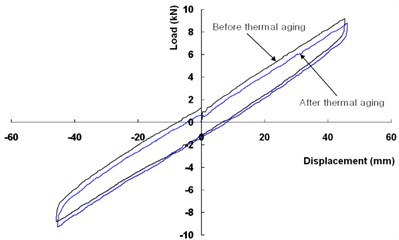a) NRB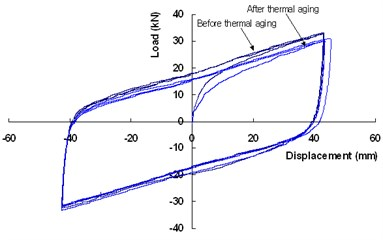b) LRB

Fig. 4Physical properties of cycle test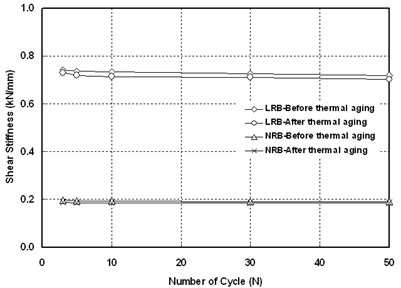a) Shear stiffness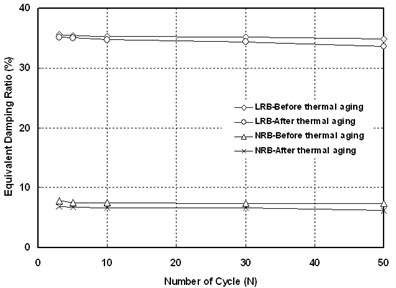b) Equivalent damping ratio

### 2.3.4. Extreme shear failure test

The extreme shear failure test was carried out in this study in order to determine the shear deformation performance of laminated rubber bearing before and after thermal aging. The test was carried out by installing one laminated rubber bearing specimen. If the test was carried out in a high speed, the shear deformation performance of the specimen may be overestimated, so the horizontal displacement was loaded up to the maximum strain with the constant wave at a low speed of 0.52 mm/sec. After installing the specimen, the maximum design compression force was loaded, and the shear displacement was applied in one direction until the laminated rubber bearing specimen failed. At this time, the test was carried out continuously until the load decreased rapidly after its maximum point, and the test was ended when it was considered that the proper failure was achieved. After the shear failure test, the shape of failed specimen is as shown in Fig. 7, and the shear failure occurred at the bottom of the specimen. The test result of each specimen is as shown in Table 4, Fig. 5 and Fig. 6. In case of lead rubber bearing, no clear strain hardening of rubber occurred, but it began to show at the shear displacement of 60 mm and the failure began to occur after 130 mm. In case of the natural rubber bearing, the strain hardening of rubber began to show at the shear displacement of approximately 100 mm and the shear failure began to occur at the shear displacement of approximately 150 mm.

Fig. 5Hysteresis curve of ultimate failure test (NRB)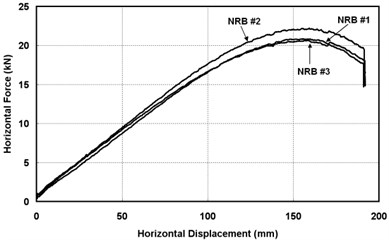a) Virgin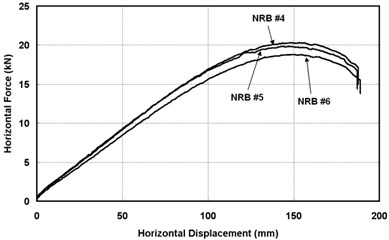b) Thermal aged

Fig. 6Hysteresis curve of ultimate failure test (NRB)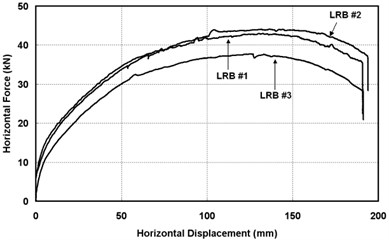a) Virgin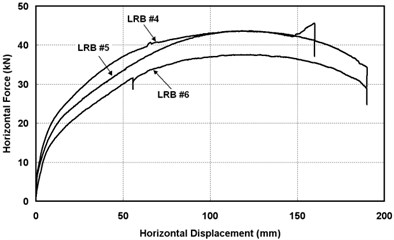b) Thermal aged

Table 4Result of ultimate failure test

 Items NRB LRB Shear strain (mm) Shear strain ratio (%) Shear strain (mm) Shear strain ratio (%) Virgin #1 157.96 170 132.91 152 #2 159.03 171 125.58 145 #3 155.51 167 136.40 157 Aged #4 148.10 159 117.91 136 #5 143.85 155 117.36 135 #6 150.51 162 118.73 137

Also, the result of extreme shear failure test before and after thermal aging showed that the shear failure displacement before aging decreased after aging. In case of the lead rubber bearing, the shear strain decreased by approximately 11 % on average, and in case of the natural rubber bearing, the shear strain decreased by approximately 6.8 %. However, in comparison between the test result and the result of previous studies, the shear strain is relatively low. According to the study conducted by Jung et al., the ultimate shear failure of lead rubber bearing and natural rubber bearing occurred at the shear strain of 500 % and 467 % respectively, which was approximately 3 times higher shear strain in comparison to this test result . Such difference in the shear strain was caused by the effect of shape factor. The basic property of rubber used in the study mentioned above and this test is same, and the primary shape factor (${S}_{1}$) for the lead rubber bearing is and the rubber bearing is 28, and the secondary shape factor (${S}_{2}$) is 7 for both cases. However, the secondary shape factor of specimen used in this test is 2.98 which is relatively low. In the studies conducted by Yasaka et al. and Oh, the critical nature test result of laminated rubber was carried out with shape factors as variables showed that as the secondary shape factor was smaller, the shear force and the shear strain significantly decreased in the event of large scale deformation, and especially in case the secondary shape factor was less than 4, the elastic restoring force decreased and the Buckling Failure occurred at the same time in the event of large scale deformation [12, 13]. This result is similar to the test result in this study.

Fig. 7Shape the ultimate failure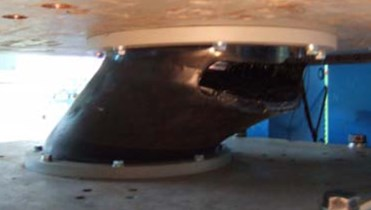a) NRB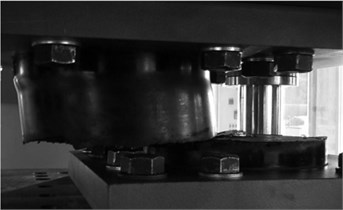b) LRB

### 2.3.5. Analysis of test results

As a result of drawing the characteristics change of laminated rubber bearing due to accelerated thermal aging through the test, all the compression stiffness, shear stiffness and equivalent damping ratio decreased after thermal aging. This result is similar to the result of studies conducted by Park et al. (2012) and KTRI . The two studies mentioned above were carried out for the lead rubber bearing using the same thermal aging conditions of 70 °C and 168 hr. According to the result of study conducted by Park et al. (2012), the compression stiffness, shear stiffness and equivalent damping ratio decreased by 3.34 %, 2.7 % and 1.9 % respectively under the same thermal aging condition. Also, according to the study carried out by KTRI, the shear stiffness increased by 1.5 % but the equivalent damping ratio decreased by 0.6 % after thermal aging.

However, according to the study carried out by Nakamura et al., as a result of test after thermal aging natural rubber bearing (NRB) for 239 days at 100 °C, the compression stiffness, shear stiffness and equivalent damping ratio increased by 17 %, 15 % and 3.2 % respectively, showing a conflicting result to this study . On the other hand, according to the study conducted by Hiroyuki et al., the test was carried out for the natural rubber bearing (NRB) as same as the study conducted by Nakamura et al. using the same thermal aging temperature of 100 °C, and as a result of test after carrying out the thermal aging for 318 hr, the compression stiffness and shear stiffness decreased by 2.0 % and 0.8 % respectively . When comparing the test conditions between Nakamura et al. and Hiroyuki et al., the same specimen as natural rubber bearing specimen and the same accelerated thermal aging temperature at 100 °C were used, but there is a significant difference in the thermal aging time as 238 days (5,712 hr) and 318 hr. This shows that there is a significant difference according to the thermal aging period even if the same accelerated thermal aging conditions are used.

## 3. Seismic performance evaluation of bridge

### 3.1. Calculation of elastic seismic forces

The target bridge is the 2@2-span continuous slab bridge located in the seismic zone I and the specifications and pier section characteristics of the target bridge are as shown in Fig. 8, Table 5 and Table 6. The seismic analysis condition is seismic rating 1, and the acceleration coefficient is 0.154 and the site coefficient is 1.2.

The modeling was carried out with the plate element for the upper structure of target bridge for analysis and the frame element for the pier. The effects of site applied to the incident seismic load and the modeling was carried out with the fixed end for the bottom of the pier. Modeling was carried out for the bearing using NL Link to match the actual behavior. The dead load of structure was calculated automatically in the program and all other dead loads such as the pavement were considered. The structure analysis model is as shown in Fig. 8 and the axial force of pier according to the dead load analysis is as shown in Table 7.

In order to calculate the sectional strength, Horizontal Displacement and effective stiffness of pier, the moment-curvature ($M-\mathrm{\varnothing }$) analysis was carried out by considering the axial force effects. The section characteristics of pier are as shown in Table 6. The Kent&Park model was used for concrete and the Menegotto-Pinto model was used for the reinforcing bar. $M-\mathrm{\varnothing }$ analysis result is as shown in Fig. 9, The effective stiffness $\left({E}_{C}\mathrm{*}{I}_{eff}\right)=\left({M}_{y}/{\mathrm{\varnothing }}_{y}\right)M-\mathrm{\varnothing }\mathrm{}$was calculated using the yield moment ${M}_{u}$ and the yield curvature ${\varnothing }_{u}$ obtained by $M-\mathrm{\varnothing }$ analysis, and the secondary moment on the effective section applied when calculating the elastic seismic force for the collapse prevent level ${I}_{eff}=\left({M}_{y}/{\mathrm{\varnothing }}_{y}\right)/\left({E}_{C}\mathrm{*}{I}_{g}\right)$ is 0.022761 m4. Here, ${E}_{c}=8,500\sqrt{{f}_{ck}+8}$ (MPa).

Table 5Parameter of bridges

 Length 4@15.0 = 60.0 m Year 1995 Rating 1 Design load DB-24 Superstructure Substructure Bridge type RC SLAB Abutment type T-type Bridge with 9.00 m Pier type $\pi$-type Slab ${f}_{ck}$ 27 MPa Footing type Steel Pile Girder ${f}_{y}$ 400 MPa Concrete ${f}_{ck}$ 24 MPa

Table 6Properties of Pier

 Items Properties Column dimension, $D$ Circle type, 1.1 m Column section ${A}_{g}$, ${I}_{g}$ 1.131 m2, 0.07187 m4 Reinforced yield stress (${f}_{y}$) 300 MPa (SD30) Concrete strength (${f}_{ck}$) 24 MPa Longitudinal reinforcement D22×25EA (= 96.775 cm2), $\rho =$1.02 % Hoop reinforcement D16 (= 1.986 cm2), $s=$125 mm Concrete cover 100 mm

Fig. 8Bridge dimensions and analysis model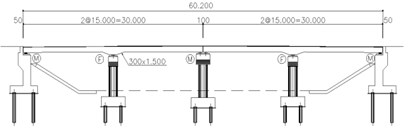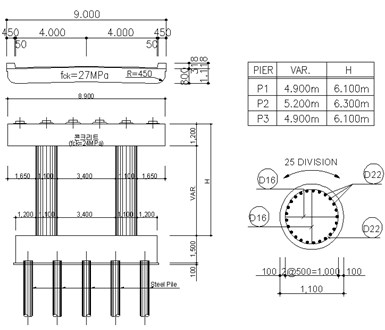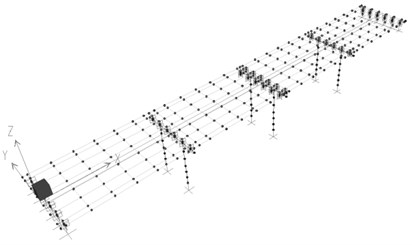The effective stiffness calculated from the section analysis of pier applied to the structural analysis model and the seismic analysis was carried out with the design response spectrum in Fig. 10 as the load. The mode number was selected to have the cumulative mass rate of vibration mode over 90 % through the eigenvalue analysis. The elastic seismic force was calculated by applying the 30 % regulation for each seismic load direction and the combined Seismic force of fixed-end pier and period vibration are as shown in Table 8.

Fig. 9Curve of M-∅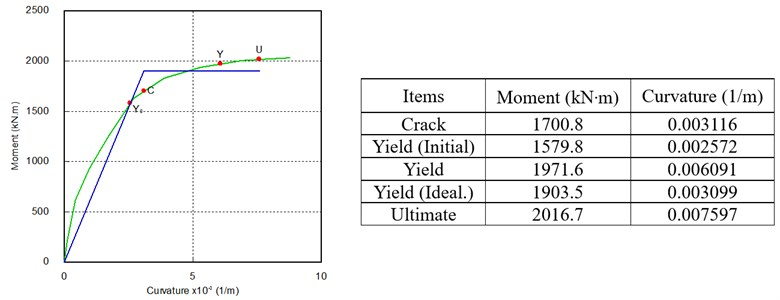Table 7Pier of the axial force due to the weight

 Items Pier top (kN) Pier lower (kN) Remarks P1 2147.7 2286.3 Fixed P2 1445.7 1598.4 Move P3 2147.7 2286.3 Fixed

Table 8Seismic forces of Pier and period vibration

 Items Axial Vertical axial Upper axial force $P$ (kN) 2146.8 Lower axials force ${P}_{Bot}$ (kN) 2263.2 Upper displacement ${∆}_{Top}$ (mm) 96.27 10.07 Upper shear force ${V}_{Top}$ (kN) 800.2 553.3 Lower shear force ${V}_{Bot}$ (kN) 808.2 571.1 Lower movement ${M}_{E}$ (kN∙m) 4830.4 1420.4 Effective height ${H}_{e}$ (m) 6.10 6.80 Period vibration $T$ (sec) 1.2769 0.3864

Fig. 10Design response spectrum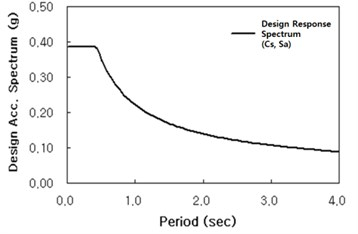### 3.2. Seismic performance evaluation of pier

The section strength means the maximum resisting capacity of framework member to the horizontal load, and the load applying point is the centroid with respect to the direction perpendicular to bridge. The Table 9 shows the Stiffness and ductility of Pier. Table 10 and Table 11 show the Pier supply capacity and the pier demand supply respectively. In case of axis direction, the displacement ductility factor is (required = 2.498 > supply = 1.488) showing that the seismic performance is insufficient, and in case of direction perpendicular to bridge, the displacement ductility factor is (required = 0.686 < supply = 1.478) showing the behavior in the elastic region. Therefore, the comparative analysis was carried out with focus on the improvement of seismic performance in the axis direction.

Table 9Stiffness and ductility of Pier

 Items Axial Vertical axial Unit ${F}_{y}={M}_{y}/{H}_{e}$ 312.0 279.9 kN ${F}_{t}={M}_{y}/{H}_{e}$ 330.6 296.6 kN ${∆}_{y}={\varnothing }_{y}*{H}_{e}/3$ 0.03844 0.04777 m ${L}_{p}=0.08*{H}_{e}+0.022{f}_{y}*{d}_{b}$ 0.633 0.689 m ${\theta }_{p}=\left({\varnothing }_{u}-{\varnothing }_{y}\right)*{L}_{p}$ 0.00285 0.00310 ${∆}_{p}=\left(\frac{{M}_{u}}{{M}_{y}}-1\right){∆}_{y}+{\theta }_{p}\left({H}_{e}-\frac{{L}_{p}}{2}\right)$ 0.0188 0.0229 m ${∆}_{u}={∆}_{y}/{∆}_{p}$ 0.0572 0.0706 m ${\mu ∆}_{c}={∆}_{u}/{∆}_{y}$ 1.488 1.478 ${V}_{c}=K*\sqrt{{f}_{ck}}*\left(0.8{A}_{g}\right)$ 1,117.4 1,117.4 kN $\stackrel{´}{D}$ 983.0 983.0 mm $\stackrel{´}{{V}_{s}=\pi *{A}_{y}*{f}_{y}*D}/\left(2*S\right)$ 702.3 702.3 kN ${V}_{P}=0.15P*D/{H}_{e}$ 58.07 52.10 ${V}_{n}={V}_{c}+{V}_{s}+{V}_{p}$ 1,877.7 1,871.7 kN

Table 10Pier supply capacity

 Items Axial Vertical axial Remark Bending strength ${F}_{n}$ (kN) 330.6 272.0 Shear strength ${V}_{n}$ (kN) 1,877.7 1,871.7 Yield displacement ${∆}_{y}$ (m) 0.0384 0.0478 Ultimate displacement ${∆}_{c}$ (m) 0.0572 0.0706 $\mu {∆}_{c}×{∆}_{y}$ Ductility ${\mu ∆}_{c}$ 1.488 1.478 Here, ${F}_{n}={F}_{y}+\frac{{F}_{f}-{F}_{y}}{{∆}_{c}-{∆}_{y}}\left({∆}_{c}-{∆}_{y}\right)$

Table 11Pier demand supply

 Items Axial Vertical Remark ${M}_{E,pd}={M}_{E}+P*{∆}_{Top}$ 5,037.1 1,442.0 Elastic seismic moment ${M}_{n}={F}_{n}×{H}_{e}$ 2,016.7 1,849.3 Nominal moment ${R}_{s}={M}_{E,pd}/{M}_{n}$ 2.498 0.780 Strength ratio ${\lambda }_{DR}$ 1.0 0.880 $\mu {∆}_{d}={\lambda }_{DR}*{R}_{S}$ 2.498 0.686 Ductility Here, $T\ge 1.25*{T}_{S}\left(=0.55\right),{\lambda }_{DR}=1.0,$$T<1.25*{T}_{S}\left(=0.55\right),{\lambda }_{DR}=\left(1-\frac{1}{{R}_{S}}\right)\frac{1.25{T}_{S}}{T}+\frac{1}{{R}_{S}}$

## 4. Seismic performance of laminated rubber bearing before and after thermal aging

### 4.1. Structural analysis modeling

In order to carry out the nonlinear time history analysis, 4 artificial earthquakes were constructed using the artificial seismic wave generation program SIMQKE under the seismic design condition by the standard design response spectrum of the highway bridge design code (2012). The seismic wave time history and seismic spectrum used are as shown in Fig. 11. The analysis model is same as shown in Fig. 8, except that all 24 bridge bearings were replaced with the lead rubber bearings and natural rubber bearings. Modeling was carried out for NL Link using the Isolator1 element which could analyze the characteristics of laminated rubber bearing. The specifications of bearing used for the Isolator1 are as shown in Table 1, and the main characteristics before and after thermal aging due to the test above are as shown in Table 12.

Table 12Properties before and after thermal aging

 Items NRB LRB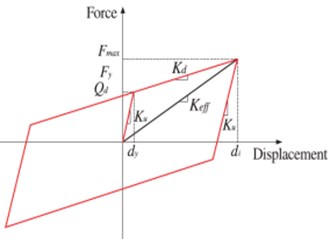Virgin Aged Virgin Aged Shear stiffness ${K}_{v}$ (kN/m) 195,233.9 190,983.3 478,193.8 462,269.6 Effective stiffness ${K}_{eff}$ (kN/m) 194.13 186.7 730.6 701.0 Characteristic Force ${Q}_{d}$ (kN) 20.42 20.42 Elastic Stiffness ${K}_{u}$ (kN/m) 3235.2 3223.6 Post-elastic Stiffness ${K}_{d}$ (kN/m) 226.5 214.9

### 4.2. Analysis of results

The primary modes shape of natural rubber bearing before and after thermal aging for the target bridge are as shown in Fig. 11. As a result of analysis, all natural periods are longer than the natural period (T) (1.277 sec) of non-isolated bridge, showing the characteristics of bridge using the seismic isolation systems properly. The natural period of natural rubber bearing before and after thermal aging was 2.822 sec and 2.772 sec respectively, showing a 1.79 % decrease after thermal aging. This is because as time passed, rubber becomes hardened so that the elasticity decreases and the hardness increases due to the characteristics of rubber aging. On the other hand, the natural period of lead rubber bearing before and after thermal aging was 1.559 and 1.588 respectively, showing a 1.65 % increase after thermal aging. This is because the shear stiffness of lead rubber bearing rather than the hardening effect due to thermal aging of rubber decreased so that the natural period became longer as the displacement of bearing itself increased.

The shear force at the bottom of pier means the shear force at the part where the column of pier and spread footing contact, and it includes the inertial force due to the dead load of upper structure delivered through the bearing and the inertial force of the column. Also, the moment at the bottom of pier is the combination of inertial force due to the dead load of upper structure delivered through the bearing and the product of column length to the inertial force of the column. The analysis result before and after thermal aging is as shown in Table 13. In case of natural rubber bearing and lead rubber bearing, the shear force, moment and the displacement at the top of pier decreased after thermal aging. As shown from the response of bearing before and after thermal aging although the level of change is low, increased displacement response means the increased energy borne by the bridge bearing, resulting in decrease in the shear force of pier.

The displacement of natural rubber bearing increased by up to 1.59 % in comparison to the displacement before thermal aging, and the displacement of lead rubber bearing increased by 0.45 %. The shear force of bearing is closely related to the economic efficiency, edge distance and height. The shear force of bearing is an element which has an influence on the size of bearing just as the vertical capacity, and if the shear force related to the displacement capacity becomes higher, the rate of material cost increase due to increase in unit size becomes higher rapidly . However, in case of seismic isolation, the safety ratio for shear force over 2 is secured in general, so increasing displacement due to the aging of bearing can be negligible.

Fig. 11Primary modes shapes and natural frequencies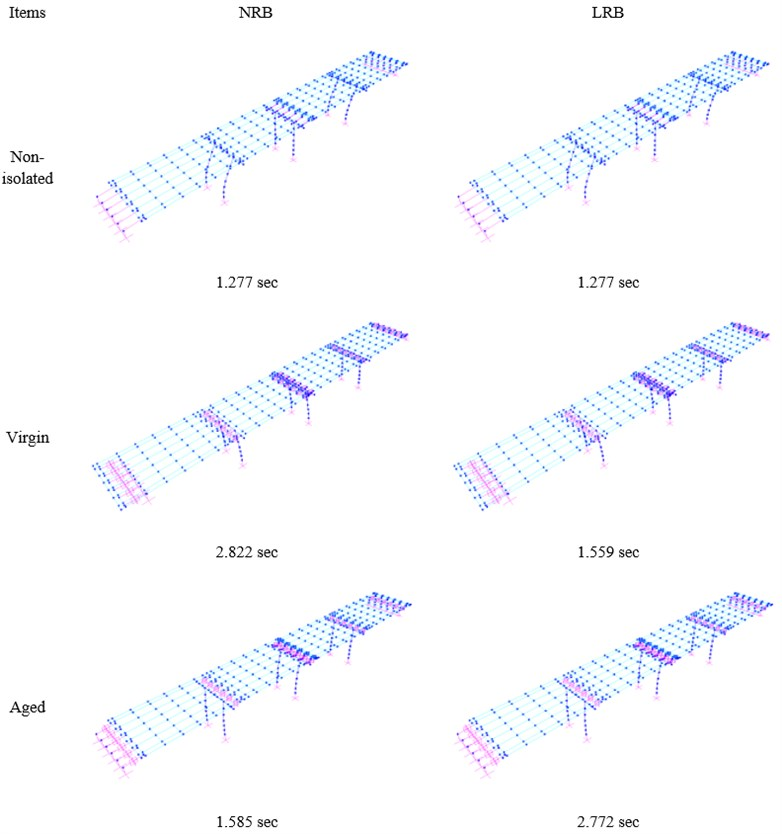The analysis result after applying the thermal aged laminated rubber bearing to an actual bridge is as shown in Table 13 and Table 14. The natural period of laminated rubber bearing according to thermal aging decreased after thermal aging in comparison to the natural period before thermal aging, and the shear force, moment of pier and the displacement at the top of pier also decreased. On the other hand, the displacement of laminated rubber bearing increased after thermal aging in comparison to the displacement of laminated rubber bearing before thermal aging. This result is similar to the result of study conducted by Kitahara . In the study conducted by Kitahara, the seismic analysis was carried out for RC bridges by considering stiffness change in case the aging of rubber bearings has been carried out for 50 years and 100 years in the area where an earthquake actually occurred. As a result, the moment and shear force of a bridge where aging has occurred for 100 years decreased by 0.8 % and 1.2 % respectively. As a result of analyzing two results above, thermal aging of the laminated rubber bearing had no significant effect on the structure system of the whole bridge.

Fig. 12Artificial seismic wave used in analysis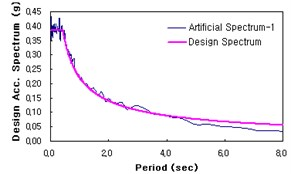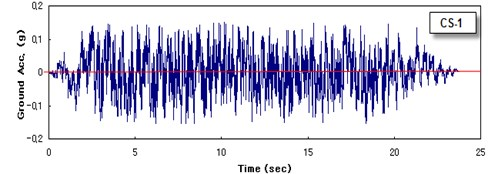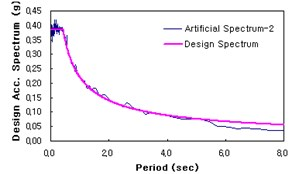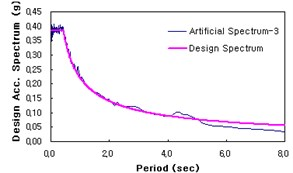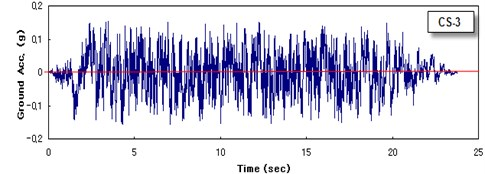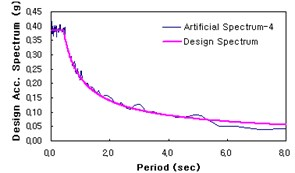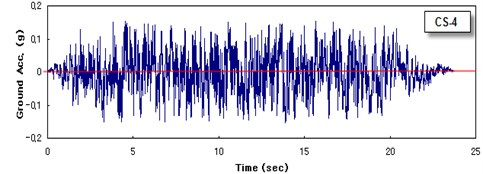Table 13Response of before and after thermal aging

 Items NRB LRB Thermal aged Changed ratio(%) Thermal aged Changed ratio(%) Before After Before After Moment P1 1,186.0 1,166.2 –1.70 833.13 827.34 –0.70 (Pier, kN∙m) P2 2,267.8 2,163.4 –4.83 1574.1 1568.63 –0.35 Shear force P1 200.52 195.36 –2.64 139.75 138.80 –0.68 (Pier, kN) P2 328.83 319.9 –2.77 238.36 237.29 –0.45 Displacement P1 23.79 23.47 –1.36 16.70 16.58 –0.72 (Pier, mm) P2 53.72 51.25 –4.81 37.18 37.06 –0.32 Displacement P1 134.9 136.9 +1.59 66.46 66.67 +0.31 (Bearing, mm) P2 134.9 137.0 +1.59 46.05 46.26 +0.45

Table 14Seismic performance of before-after thermal aging

 Items NRB LRB Period(sec) Shear force(kN) Displacement(mm) Period(sec) Shear force(kN) Displacement(mm) Supply capacity – 330.6 57.2 – 330.6 57.2 Non-isolated 1.277 808.2 96.27 1.277 808.2 96.27 Before aged 2.772 200.5 23.79 1.559 139.75 16.70 After aged 2.822 195.4 23.47 1.585 138.80 16.58 Changed ratio (%) –1.79 –2.64 –1.36 +1.65 –0.68 –0.72

## 5. Conclusion

In this study, the characteristics of laminated rubber bearing before and after thermal aging were analyzed by applying accelerated thermal aging to the laminated rubber bearing, and the influence of thermal aging of bearing on the seismic performance of bridge was compared and the following conclusion was obtained.

1) As a result of compression test before and after thermal aging, the compression stiffness decreased by approximately 2-3 % after aging. Especially, the horizontal displacement of lead rubber bearing decreased due to the compression load and its slope increased. And, as a result of examining changes in the shear stiffness and equivalent damping ratio due to thermal aging through the compression-shear test, the shear stiffness and equivalent damping ratio decreased in all specimens, and especially the lead rubber bearing showed a relatively significant decrease in the shear stiffness. Also, as a result of carrying out the cyclic loading test for 50 times under the same conditions as above, the shear stiffness decreased by 2.7-3.6 %, and the equivalent damping ratio decreased by 1.9 % in case of lead rubber bearing and 10.9 % in case of natural rubber bearing, indicating that the natural rubber bearing was somewhat affected by heat. As a result of extreme shear failure test before and after thermal aging, the shear strain decreased after aging.

2) As a result of carrying out the seismic performance analysis of RC bridge by applying the properties of laminated rubber bearing before and after thermal aging, the characteristics of bridge using the seismic isolation systems were shown properly. In case of laminated rubber bearing before and after thermal aging, the natural period, the shear force of pier, moment and the displacement at the top of pier decreased after aging. On the other hand, the displacement of laminated rubber bearing increased after thermal aging in comparison to the displacement of laminated rubber bearing before thermal aging. The seismic performance of bridge may vary to a certain degree according to the capacity and arrangement of laminated rubber bearing. However, there was no significant change in the seismic performance of bridge according to the thermal aging characteristic change of laminated rubber bearing in the target specimen or bridge condition in this study. In consideration of safety rate of bridge bearing design, thermal aging of the laminated rubber bearing will have no significant effect on the structure system of the whole bridge.

3) Studies regarding the thermal aging of laminated rubber bearing generally focus on the accelerated thermal temperature condition. However, as a result of comparative analysis between this study and precedent studies, under the same aging temperature, as rubber is exposed to the aging condition for longer time, the hardening speed of rubber becomes faster, increasing the stiffness of rubber so that it is expected that the thermal aging characteristics of laminated rubber bearing will appear clearly.

4) In order to identify the effect of thermal aging on the laminated rubber bearing and the seismic performance of bridge more clearly, it is necessary to carry out the test under more severe accelerated thermal temperature and aging time conditions. It is also necessary to consider the effects according to repetitive temperature changes under the atmospheric temperature condition for a long period of time.

06 December 2015
Accepted
18 August 2016
Published
30 September 2016
SUBJECTS
Seismic engineering
Keywords
natural rubber bearing
isolator
thermal aging
seismic performance
Acknowledgements

This research was supported by Yeungnam University Research Grant in 2015.